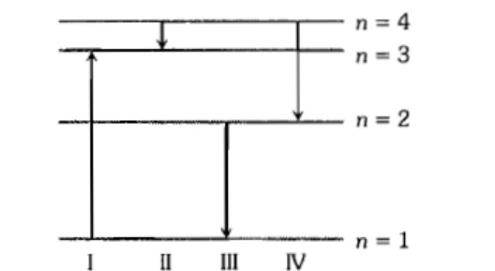The diagram shows the energy levels for an electron in a certain atom. Which transition shown represents the emission of a photon with the most energy?1. I

2. II

3. III

4. IV

Subtopic:  Spectral Series |
To view explanation, please take trial in the course below.
NEET 2022 - Target Batch - Aryan Raj Singh

Difficulty Level:

The kinetic energy of the electron in an orbit of radius r in the hydrogen atom is (e = electronic charge)

1. $\frac{k{e}^{2}}{{r}^{2}}$

2. $\frac{k{e}^{2}}{2r}$

3. $\frac{k{e}^{2}}{r}$

4. $\frac{k{e}^{2}}{2{r}^{2}}$

Subtopic:  Bohr's Model of Atom |
To view explanation, please take trial in the course below.
NEET 2022 - Target Batch - Aryan Raj Singh

Difficulty Level:

Whenever a hydrogen atom emits a photon in the Balmer series:

1. It may emit another photon in the Balmer series

2. It must emit another photon in the Lyman series

3. It may emit another photon in the Paschen series

4. It need not emit any more photon

Subtopic:  Spectral Series |
To view explanation, please take trial in the course below.
NEET 2022 - Target Batch - Aryan Raj Singh

Difficulty Level:

The ionisation potential of hydrogen atom is

1. 13.60 volt

2. 8.24 volt

3. 10.36 volt

4. 14.24 bolt

Subtopic:  Bohr's Model of Atom |
To view explanation, please take trial in the course below.
NEET 2022 - Target Batch - Aryan Raj Singh

Difficulty Level:

Which source is associated with a line emission spectrum?

1. Electric fibre

2. Neon street Sign

3. Red traffic light

4. Sun

Subtopic:  Spectral Series |
To view explanation, please take trial in the course below.
NEET 2022 - Target Batch - Aryan Raj Singh

Difficulty Level:

Ionisation potential of hydrogen atom is 13.6 eV. Hydrogen atoms in the ground state are excited by monochromatic radiation of photon energy 12.1 eV. According to Bohr's theory, the spectral lines emitted by hydrogen will be:

1. two

2. three

3. four

4. one

Subtopic:  Spectral Series |
To view explanation, please take trial in the course below.
NEET 2022 - Target Batch - Aryan Raj Singh

Difficulty Level:

Frequency of the series limit of Balmer series of hydrogen atom in terms of Rydberg constant R and velocity of light C is:

1. $\frac{RC}{4}$

2. RC

3. $\frac{4}{RC}$

4. 4RC

Subtopic:  Spectral Series |
To view explanation, please take trial in the course below.
NEET 2022 - Target Batch - Aryan Raj Singh

Difficulty Level:

Orbital acceleration of electron is

1. $\frac{{n}^{2}{h}^{2}}{4{\mathrm{\pi }}^{2}{\mathrm{m}}^{2}{\mathrm{r}}^{3}}$

2. $\frac{{n}^{2}{h}^{2}}{4{n}^{2}{\mathrm{r}}^{3}}$

3. $\frac{4{n}^{2}{h}^{2}}{{\mathrm{\pi }}^{2}{\mathrm{m}}^{2}{\mathrm{r}}^{3}}$

4. $\frac{4{n}^{2}{h}^{2}}{4{\mathrm{\pi }}^{2}{\mathrm{m}}^{2}{\mathrm{r}}^{3}}$

Subtopic:  Bohr's Model of Atom |
To view explanation, please take trial in the course below.
NEET 2022 - Target Batch - Aryan Raj Singh

Difficulty Level:

Consider the spectral line resulting from transition n = 2 to n = 1 in the atoms and ions given below.  The shorterst wavelength is given by

1. Hydrogen atom

2. Deuterium

3. Singly ionised helium

4. Doubly ionised lithium

Subtopic:  Spectral Series |
To view explanation, please take trial in the course below.
NEET 2022 - Target Batch - Aryan Raj Singh

Difficulty Level:

An $\alpha -$particle of energy 5 MeV is scattered through $180°$ by a fixed uranium nucleus. The distance of closest approach is of the order

1.

2.

3.

4.

Subtopic:  Various Atomic Models |
To view explanation, please take trial in the course below.
NEET 2022 - Target Batch - Aryan Raj Singh

Difficulty Level: